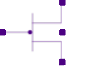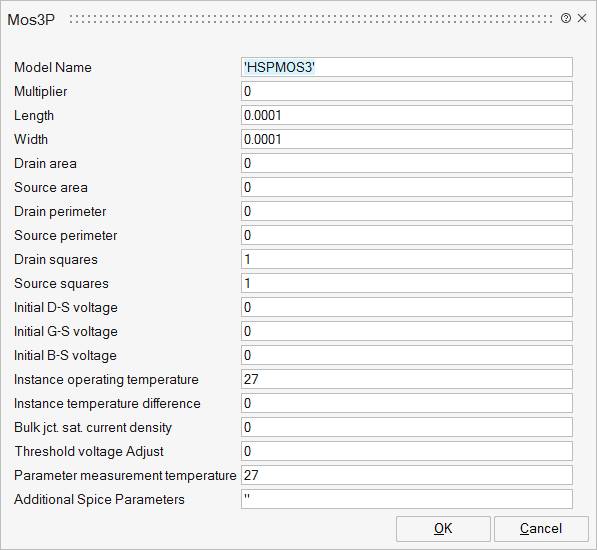# Mos3P

Level 3 MOSfet (a derived level 1 model)Analog/Basic

## Description

This is a MOS Level 3 and the formulations are derived from the model 1 and take into account a set of physical limitations in a semi-empirical way.

The model introduces the saturation voltage

The characteristics described below apply for a NMOS (see Mos3N). For a PMOS, the Ids and Vds axis have a negative sign.## ParametersNameLabelDescriptionData TypeValid Values

sp_mname

Model Name

Name of the MOS model

String

sp_m

Multiplier

Multiplier for setting m mosfets in parallel

Scalar

sp_l

Length

Channel length (meter)

Scalar

sp_w

Width

Channel width (meter)

Scalar

Drain area

Drain area (square meter)

Scalar

sp_as

Source area

Source area (square meter)

Scalar

sp_pd

Drain perimeter

Drain perimeter (meter)

Scalar

sp_ps

Source perimeter

Source perimeter (meter)

Scalar

sp_nrd

Drain squares

Equivalent number of squares of the drain diffusion

Scalar

sp_nrs

Source squares

Equivalent number of squares of the source diffusion

Scalar

sp_icvds

Initial D-S voltage

Initial drain source voltage (volts)

Scalar

sp_icvgs

Initial G-S voltage

Initial gate source voltage (volts)

Scalar

sp_icvbs

Initial B-S voltage

Initial bulk source voltage (volts)

Scalar

sp_temp

Instance operating temperature

The temperature at which the mosfet is operating (degrees Celsius)

Scalar

sp_dtemp

Instance temperature difference

Temperature variation relative to the simulation temperature (Degress Celsius)

Scalar

sp_js

Bulk jct. sat. current density

Bulk junction saturation current density

Scalar

sp_delvto

The threshold voltage is defined as the gate voltage above which the transistor becomes conductive due to an inversion of a thin layer (volts)

Scalar

sp_tnom

Parameter measurement temperature

This parameter describes the process of measuring a current local temperature

Scalar

sp_Spice

For more details, see Additional Spice Parameters.

String

## Ports

NameTypeDescriptionIO TypeNumber

Drain

implicit

Drain node

1

Gate

implicit

Gate node

2

Source

implicit

Source node

3

Bulk

implicit

Bulk node

4